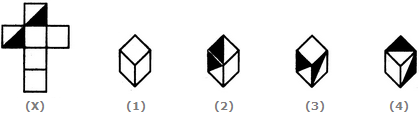# Non Verbal Reasoning - Cubes and Dice - Discussion

### Discussion :: Cubes and Dice - Section 1 (Q.No.2)

The sheet of paper shown in the figure (X) given on the left hand side, in each problem, is folded to form a box. Choose from amongst the alternatives (1), (2), (3) and (4), the boxes that are similar to the box that will be formed.

2.

Choose the box that is similar to the box formed from the given sheet of paper (X).[A]. 1 and 4 only [B]. 3 and 4 only [C]. 1 and 2 only [D]. 2 and 3 only

Explanation:

The fig. (X) is similar to the Form I. So, when the sheet shown in fig. (X) is folded to form a cube then one of the two half-shaded faces lies opposite to one of the blank faces and the other half-shaded face lies opposite to another blank face. The two remaining blank faces lie opposite to each other. Thus, both the cubes shown in figures (1).and (4) can be formed when the sheet shown in fig. (X) is folded. Also, though the cubes shown in figures (2) and (3) have faces that can appear adjacent to each other but the cube formed by folding the sheet in fig. (X) cannot be rotated to form either of the two. Hence, the cubes in figures (2) and (3) cannot be formed.

 Dhivya said: (Jan 9, 2012) I can't get these type of sums. Can anyone explain?

 Savita said: (Mar 29, 2015) I did get that shaded parts explain properly.

 Manuj Shrama said: (May 20, 2015) Hi, not able to get how blank one is correct.

 Sushma said: (Aug 6, 2015) I dint get this type of problems can anyone help me.

 Rahul said: (Aug 19, 2015) I am unable to solve this type questions. How does rotation of dice work please explain it?

 Keerthi said: (Aug 22, 2016) Please can anyone explain it.

 Nolem said: (Sep 11, 2016) The black triangles are connected thru there pointed angles. See figure 4. then analyze the figure (x). Figure it out why they'll come to the answer option A.

 Ravi Nikam said: (Jun 24, 2017) Given answer is right. Beacause option 2 and 3 triangle is not given in proper ways so given answer is true.

 Tannvi said: (Jun 20, 2020) Didn't get how the option 1 is correct? Please explain.

 Perarasu said: (Aug 25, 2020) I am not understanding this, please anyone explain me in detail.

 Rajeshree N Donuur said: (Dec 10, 2020) Good question. Thanks.